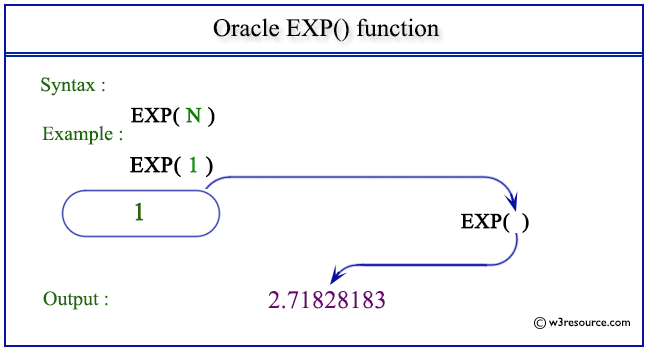# Oracle EXP() function

## Description

The Oracle EXP() function is used to get the value of the base of natural logarithm number e, raised to the power of a number specified as argument, where e = 2.71828183...
The function takes any numeric or nonnumeric data type (can be implicitly converted to a numeric data type) as an argument.
If the argument is BINARY_FLOAT, then the function returns BINARY_DOUBLE. Otherwise, the function returns the same numeric data type as the argument.

Syntax:

EXP(N);

Parameters:

Name Description
N A number.

Pictorial Presentation of EXP() functionExample:

The statement below will return the value of the natural logarithm base number e raised to the power of the number 1.

``````SELECT EXP(1) FROM dual;
```
```

Here is the result.

```    EXP(1)
----------
2.71828183
```

Example: EXP() function with negative value

The statement below will return the value of the natural logarithm base number e raised to the power of the number -1.

``````SELECT EXP(-1) FROM dual;
```
```

Here is the result.

```   EXP(-1)
----------
.367879441
```

Previous: COSH
Next: FLOOR

﻿